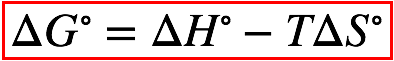# Problem: For the reaction Fe3O4 (s) + 4H2 (g) → 3Fe (s) + 4H2O (g) ΔH° = 151.2 kJ and ΔS° = 169.4 J/K The standard free energy change for the reaction of 2.33 moles of Fe3O4(g) at 311 K, 1 atm would be ___________ kJ.This reaction is (reactant, product) ____________ favored under standard conditions at 311 K.Assume that ΔH° and ΔS° are independent of temperature.

###### FREE Expert Solution
98% (309 ratings)
###### FREE Expert Solution

We’re being asked to determine the standard free energy change (ΔG) for the reaction of 2.33 mol Fe3O4. The given reaction is:

Fe­3O4(s) + 4 H2(g)  3 Fe(s) + 4 H2O(g); ΔH˚ = 151.2 kJ, ΔS˚ = 169.4 J/K

In the balanced chemical equation, 1 mole of Fe3O4 reacts. We can calculate for ΔG˚ of 1 mole of Fe3O4 using the following equation:98% (309 ratings)###### Problem Details

For the reaction

Fe3O4 (s) + 4H2 (g) → 3Fe (s) + 4H2O (g)

ΔH° = 151.2 kJ and ΔS° = 169.4 J/K

The standard free energy change for the reaction of 2.33 moles of Fe3O4(g) at 311 K, 1 atm would be ___________ kJ.

This reaction is (reactant, product) ____________ favored under standard conditions at 311 K.

Assume that ΔH° and ΔS° are independent of temperature.

What scientific concept do you need to know in order to solve this problem?

Our tutors have indicated that to solve this problem you will need to apply the Gibbs Free Energy concept. You can view video lessons to learn Gibbs Free Energy. Or if you need more Gibbs Free Energy practice, you can also practice Gibbs Free Energy practice problems.

What is the difficulty of this problem?

Our tutors rated the difficulty ofFor the reaction Fe3O4 (s) + 4H2 (g) → 3Fe (s) + 4H2O (g) ΔH...as high difficulty.

How long does this problem take to solve?

Our expert Chemistry tutor, Dasha took 5 minutes and 15 seconds to solve this problem. You can follow their steps in the video explanation above.

What professor is this problem relevant for?

Based on our data, we think this problem is relevant for Professor Tang's class at USF.